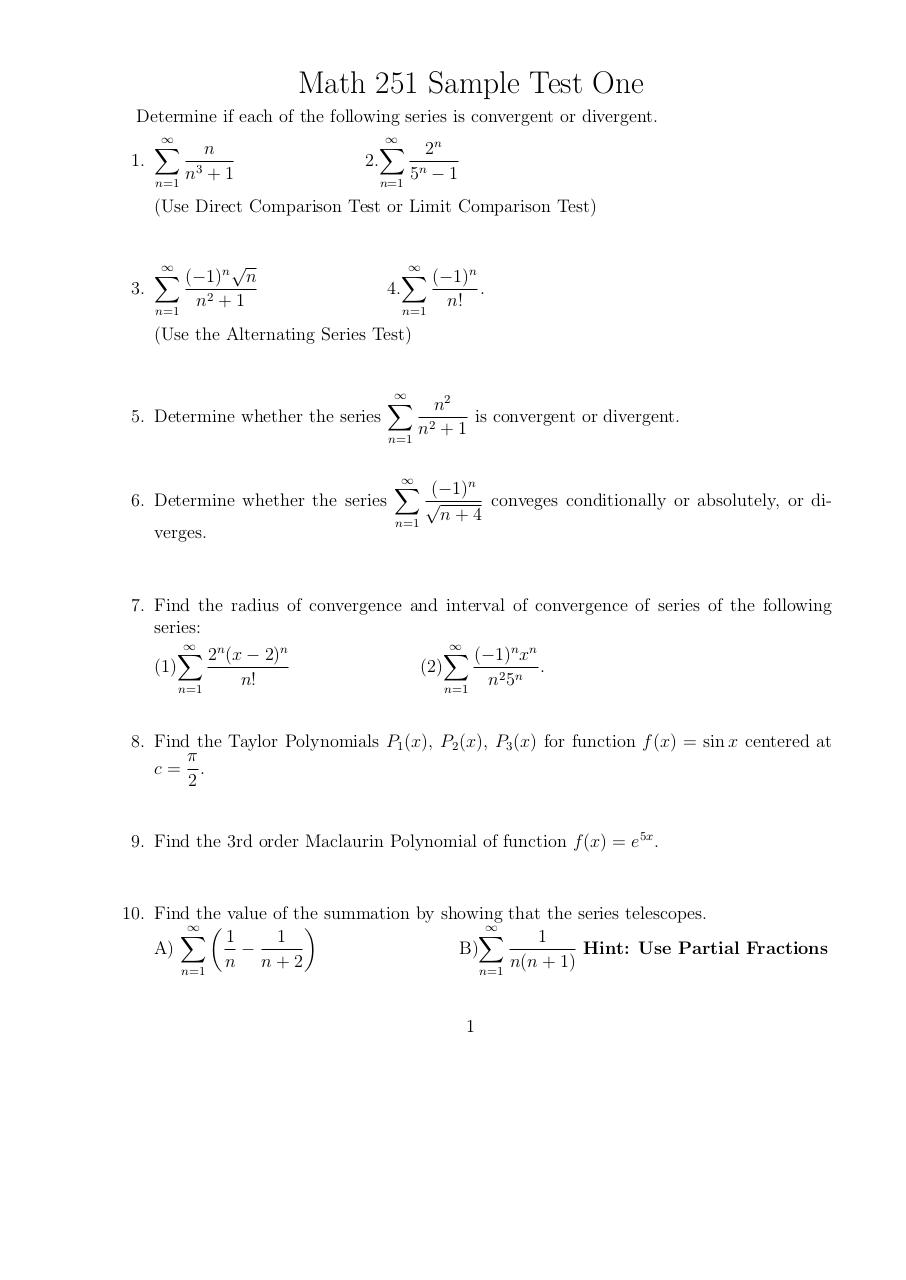# Math 251 Sample Test 1 .pdf

### File information

Original filename: Math 251 Sample Test 1.pdf

This PDF 1.4 document has been generated by TeX output 2014.02.28:0952 / MiKTeX-dvipdfmx (20120420), and has been sent on pdf-archive.com on 05/03/2014 at 00:21, from IP address 24.53.x.x. The current document download page has been viewed 647 times.
File size: 21 KB (1 page).
Privacy: public file

Math 251 Sample Test 1.pdf (PDF, 21 KB)

### Document preview

Math 251 Sample Test One
Determine if each of the following series is convergent or divergent.

2n
n
2.
1.
n3 + 1
5n − 1
n=1
n=1
(Use Direct Comparison Test or Limit Comparison Test)

3.

(−1)n n
n=1

n2 + 1

(−1)n
4.
.
n!
n=1

(Use the Alternating Series Test)

5. Determine whether the series

n=1

n2
is convergent or divergent.
n2 + 1

(−1)n

6. Determine whether the series
conveges conditionally or absolutely, or din+4
n=1
verges.

7. Find the radius of convergence and interval of convergence of series of the following
series:

2n (x − 2)n
(−1)n xn
(1)
(2)
.
n!
n2 5n
n=1
n=1
8. Find the Taylor Polynomials P1 (x), P2 (x), P3 (x) for function f (x) = sin x centered at
π
c= .
2
9. Find the 3rd order Maclaurin Polynomial of function f (x) = e5x .

10. Find the value of the summation by showing that the series telescopes.
)
∞ (

1
1
1

Hint: Use Partial Fractions
A)
B)
n
n
+
2
n(n
+
1)
n=1
n=1
1#### HTML Code

Copy the following HTML code to share your document on a Website or Blog

#### QR Code### Related keywords